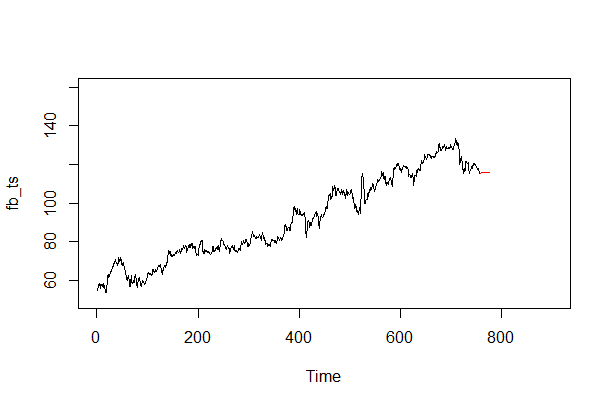# Automatic Identification of Model Using auto.arima() Function in R

### auto.arima() Function

R also has a package called forecast, which contains many forecasting functions for time series and linear models. It also contains a very useful function called auto.arima, which returns the best ARIMA model according to either AIC, AICc or BIC value. The function conducts a search over possible models within the order constraints provided.

Let's try the auto.arima function on our time series.

> fb\_fit\_auto <- auto.arima(fb\_ts)
> fb\_fit\_auto
Series: fb\_ts
ARIMA(1,1,4) with drift
Coefficients:
ar1     ma1      ma2      ma3      ma4   drift
-0.8405  0.8626  -0.0123  -0.1632  -0.1768  0.0800
s.e.   0.1080  0.1111   0.0488   0.0509   0.0381  0.0478
sigma^2 estimated as 2.581:  log likelihood=-1426.29
AIC=2866.58   AICc=2866.73   BIC=2898.97
>


The auto.arima function suggests the best fit model as ARIMA(1,1,4) with drift.

The following code creates the forecast for the FB stock prices using the suggested model:

fb\_fit <- arima(fb\_ts, order = c(1, 1, 4))
fb\_forecast <- predict(fb\_fit , n.ahead = 20)
fb\_forecast\_values <- fb\_forecast\$pred
plot.ts(fb\_ts, xlim = c(0, 900), ylim = c(50,160))
points(fb\_forecast\_values , type = "l", col = 2)